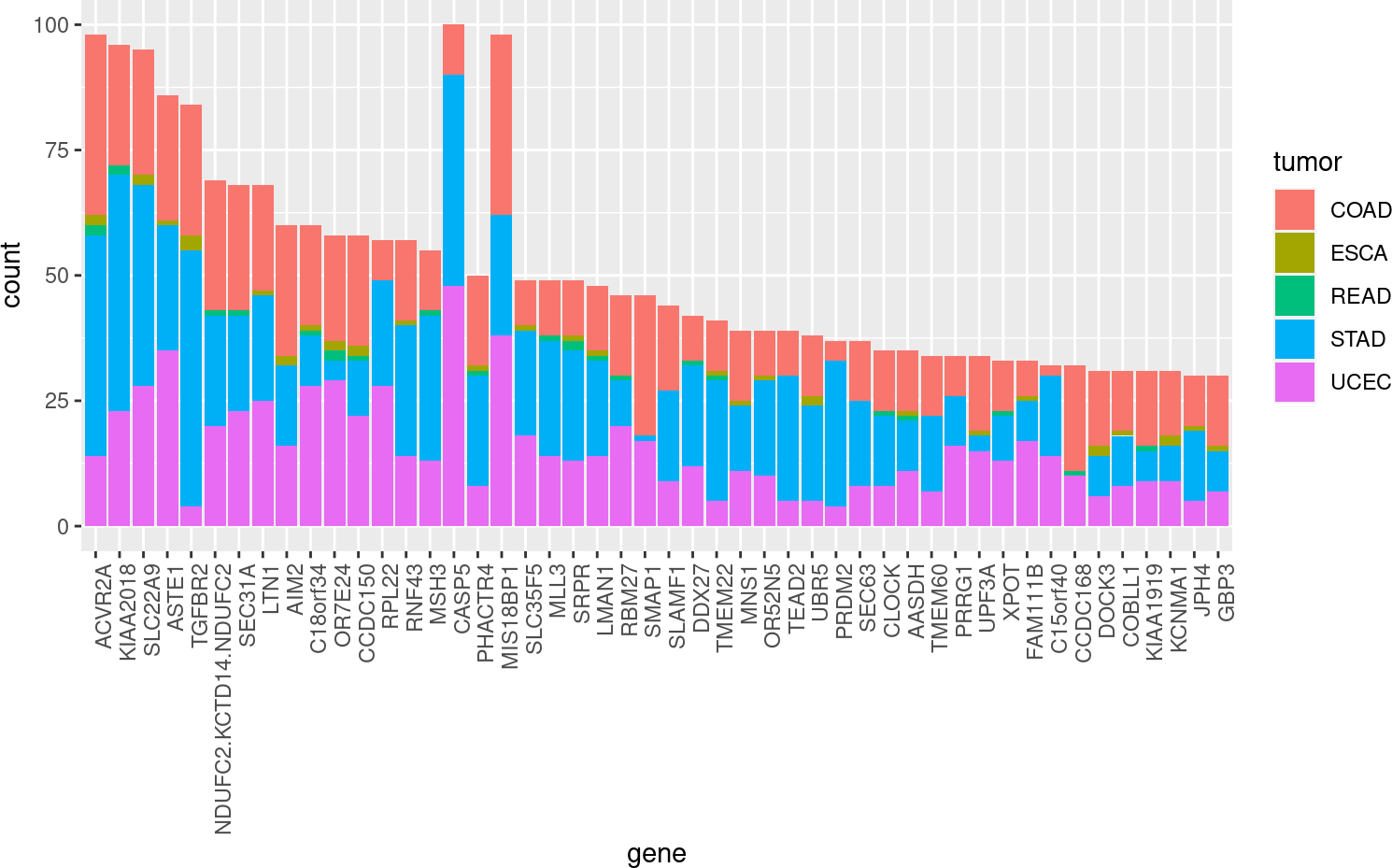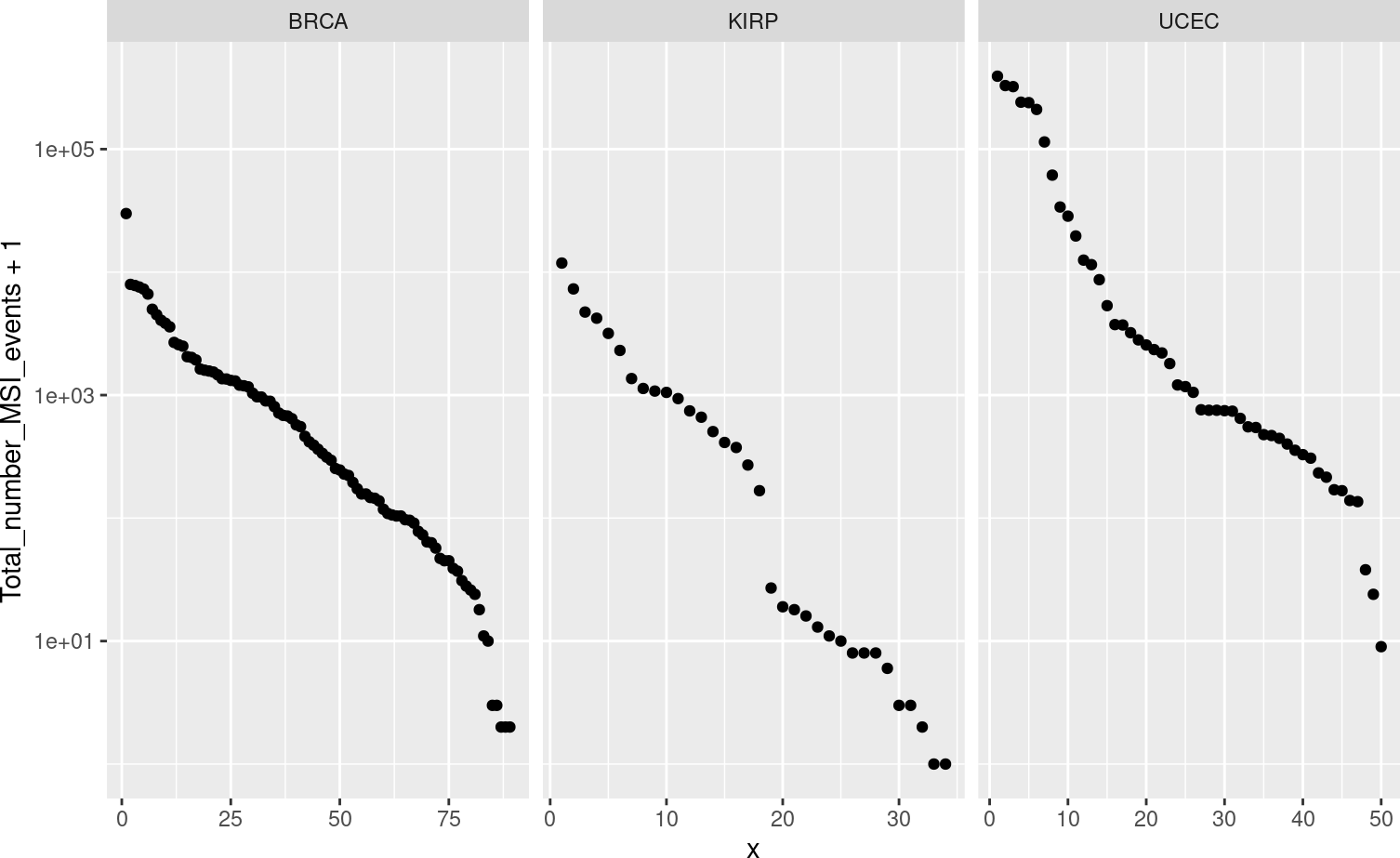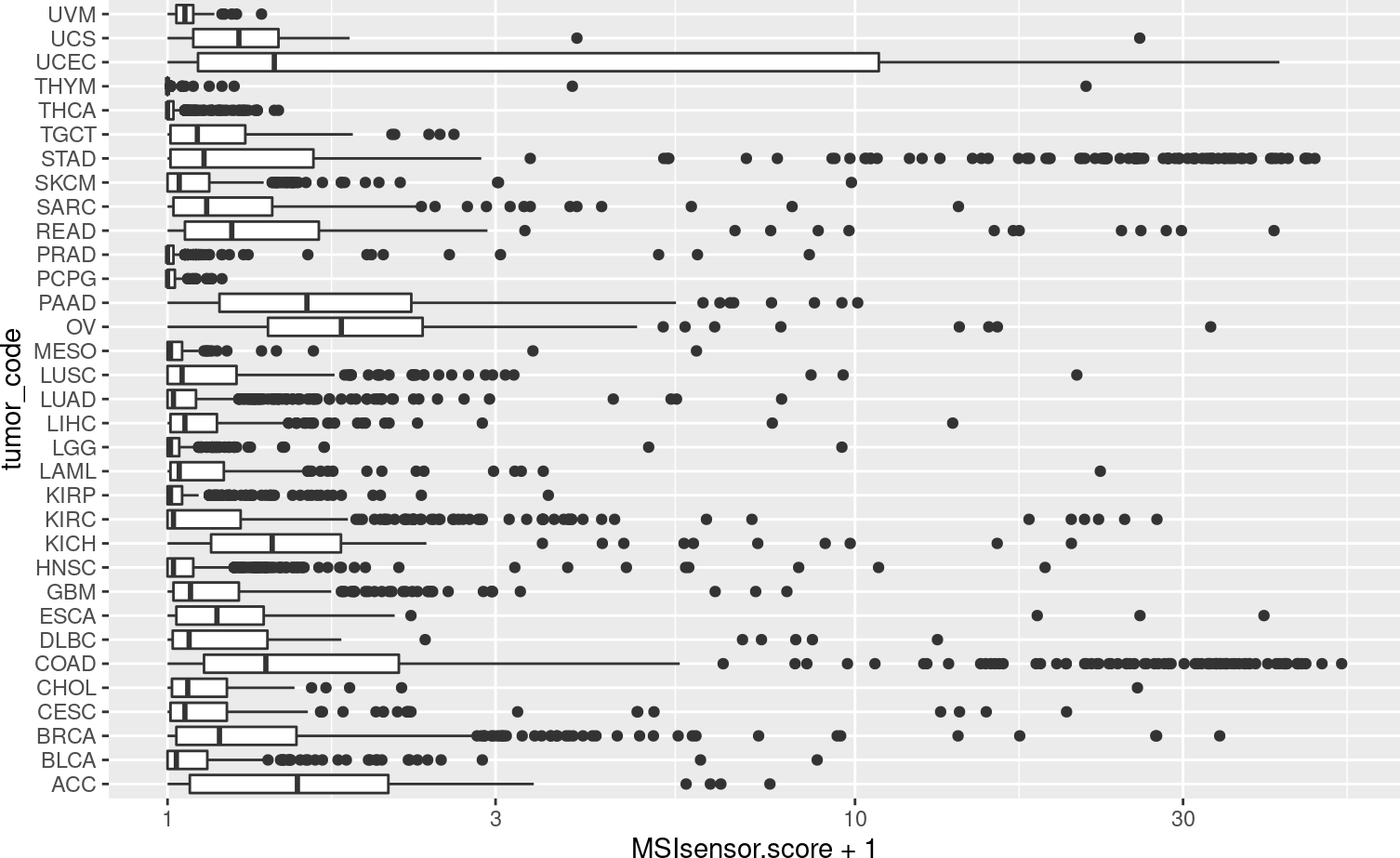# 1 Introduction

Microsatellite instability (MSI) is a feature of tumor genomes that has long been studied. A basic source of interest in MSI is the observation that tumors exhibiting MSI are often less aggressive. One theory is that these tumors produce neoantigens that are more likely to stimulate immune responses that reduce risk of metastasis.

This package provides data structures based on tables published with recent studies of MSI in TCGA samples.

# 2Cortes-Ciriano et al. 2017

Three supplemental tables have been transformed to SummarizedExperiment instances. These are devoted to gene-centric enumeration of MSI events in CDS (molpo_CDS), 3’UTR (molpo_3utr), and 5’UTR (molpo_5utr).

suppressPackageStartupMessages({
library(BiocOncoTK)
library(ggplot2)
})
molpo_CDS
## class: RangedSummarizedExperiment
## dim: 6441 190
## assays(1): sputnik_based
## rownames(6441): TNFRSF9_7998253 TCEB3_24078403 ... GPR112_135431207
##   HCFC1_153219645
## rowData names(2): gene full_rn
## colnames(190): TCGA-B5-A0JZ TCGA-D1-A175 ... TCGA-WS-AB45 TCGA-NH-A5IV
## colData names(1): tumor_type

With the following code we approximately reproduce Figure 2a of the paper. We sorted by ‘locus-specific’ MSI recurrence, then grouped by gene symbol. CASP5 occurs twice in Figure 2a; the figure below sums all the events tabulated for CASP5. How to dissect the annotation of MSI enumeration for this gene is not immediately clear. The same situation arises with gene MAK16 for 3’UTR.

library(SummarizedExperiment)
sev = apply(assay(molpo_CDS),1,sum)
litass = assay(molpo_CDS[names(sort(sev,decreasing=TRUE)[1:50]),])
dd = data.frame(t(litass), tumor=molpo_CDS$tumor_type) library(dplyr) library(magrittr) names(dd) = gsub("_.*", "", names(dd)) library(reshape2) ddm = melt(dd) ## Using tumor as id variables names(ddm) = "gene" names(ddm) = "msi_cds" if (.Platform$OS.type != "windows") {
ggplot(ddm %>% filter(msi_cds>0), aes(x=gene,fill=tumor)) + geom_bar() +
theme(axis.text.x = element_text(angle = 90, hjust = 1))
}Figure 3a of the paper illustrates variation within and between tumor types in total numbers of MSI events detected in whole genome sequences. The gestalt of this figure can be obtained through the following code:

lit = molpo_WGS[1,molpo_WGS$tumor_type %in% c("UCEC", "BRCA", "KIRP")] myd = data.frame(totmsi=t(assay(lit[1,])), tumor=lit$tumor_type)
ss = split(myd, myd$tumor) ss[]$x = 1:nrow(ss[])
ss[]$x = 1:nrow(ss[]) ss[]$x = 1:nrow(ss[])
ssd = do.call(rbind,ss)
if (.Platform$OS.type != "windows") { ggplot(ssd, aes(y=Total_number_MSI_events+1,x=x)) + geom_point() + facet_grid(.~tumor, scales="free_x")+ scale_y_log10() }# 3Ding et al. 2018 The MSIsensor scores from the paper of Ding et al. are provided in a simple data.frame. The gestalt of their Figure 3C can be obtained via: data(MSIsensor.10k) data(patient_to_tumor_code) names(MSIsensor.10k) = "patient_barcode" mm = merge(MSIsensor.10k, patient_to_tumor_code) if (.Platform$OS.type != "windows") {
ggplot(mm, aes(y=MSIsensor.score+1, x=tumor_code)) +
geom_boxplot() + coord_flip() + scale_y_log10()
}